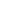# Use rectangular arrays to investigate odd and even numbers

EngageNY 60 min(s)
In this lesson, students use arrays to investigate even and odd numbers. They solidify the understanding that an odd number is either 1 more or 1 less than an even number and that it cannot be decomposed into two equal groups or groups of two, although it may be decomposed into more than two groups. They discover that the sum of two even numbers is even, and the sum of two odd numbers is even. They also discover that the sum of an odd number and an even number is odd.